2017-03-18
Thank you for participating to Moku-Moku-Meeting at PCN Akihabara Assemblage!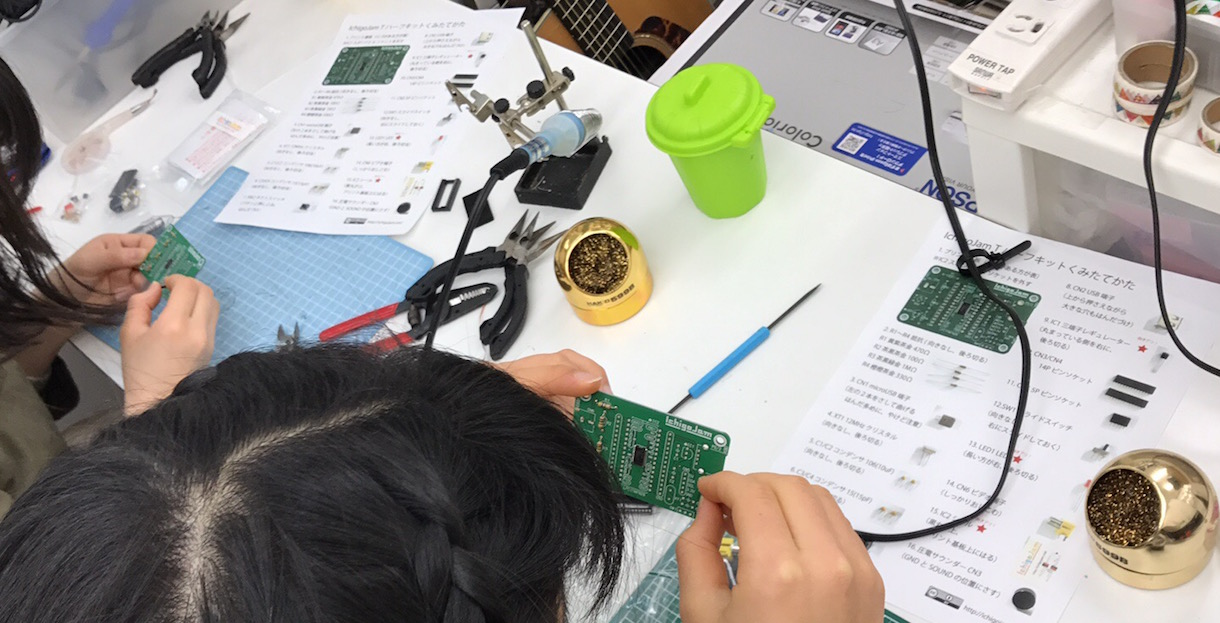Junior high school girls made the IchigoJam kit!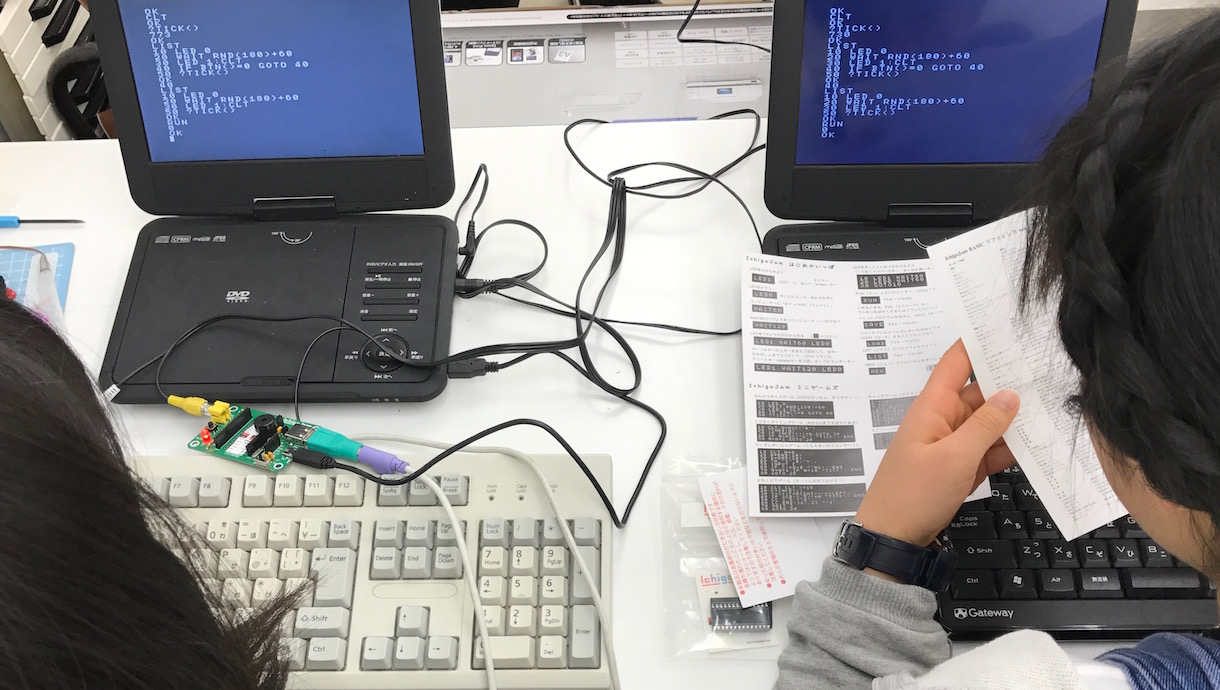Let's discovery the programming world!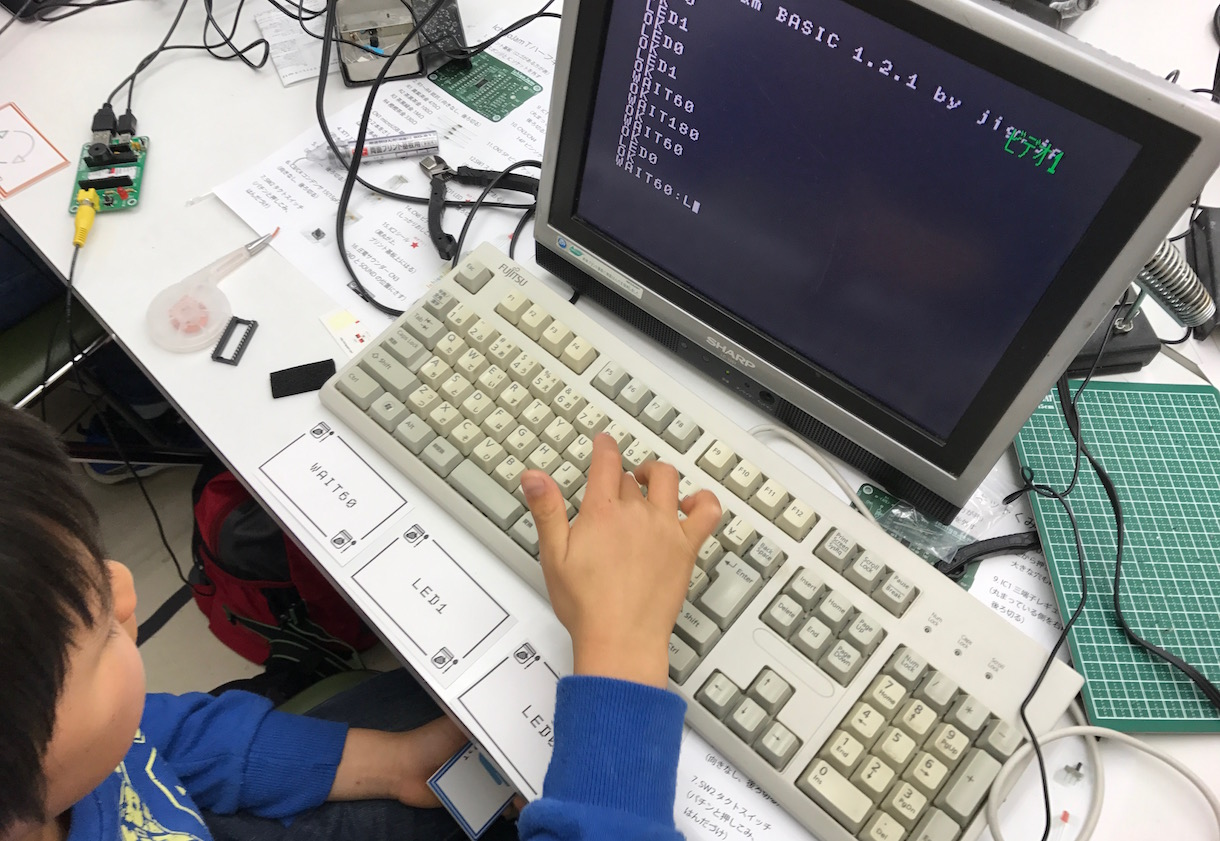The first step with CutleryCard! You did it!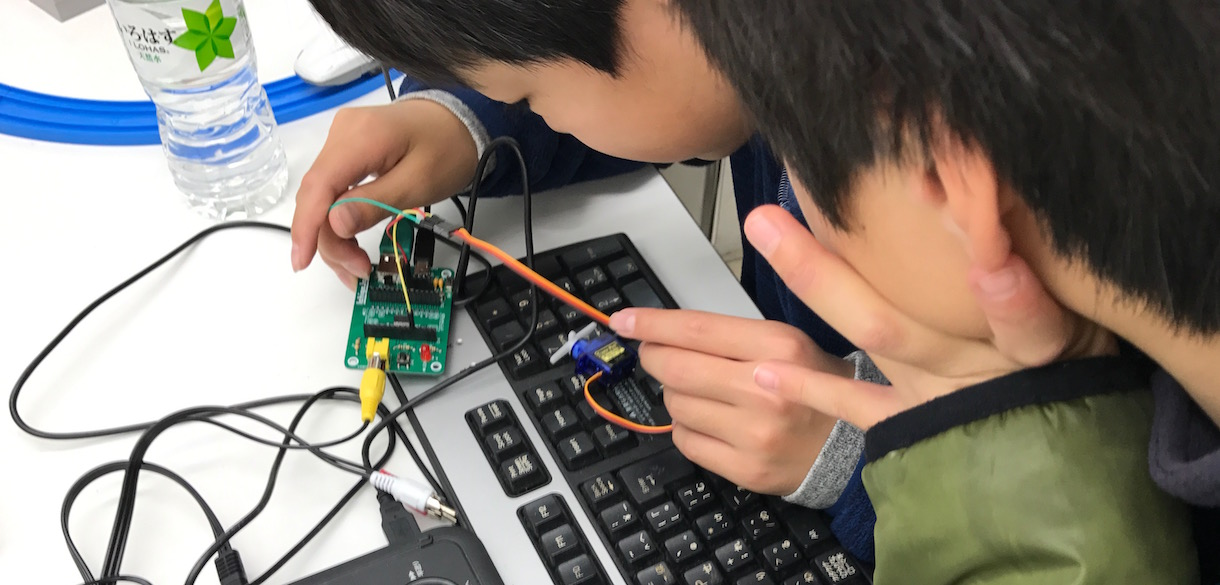The first try to move the servo motor!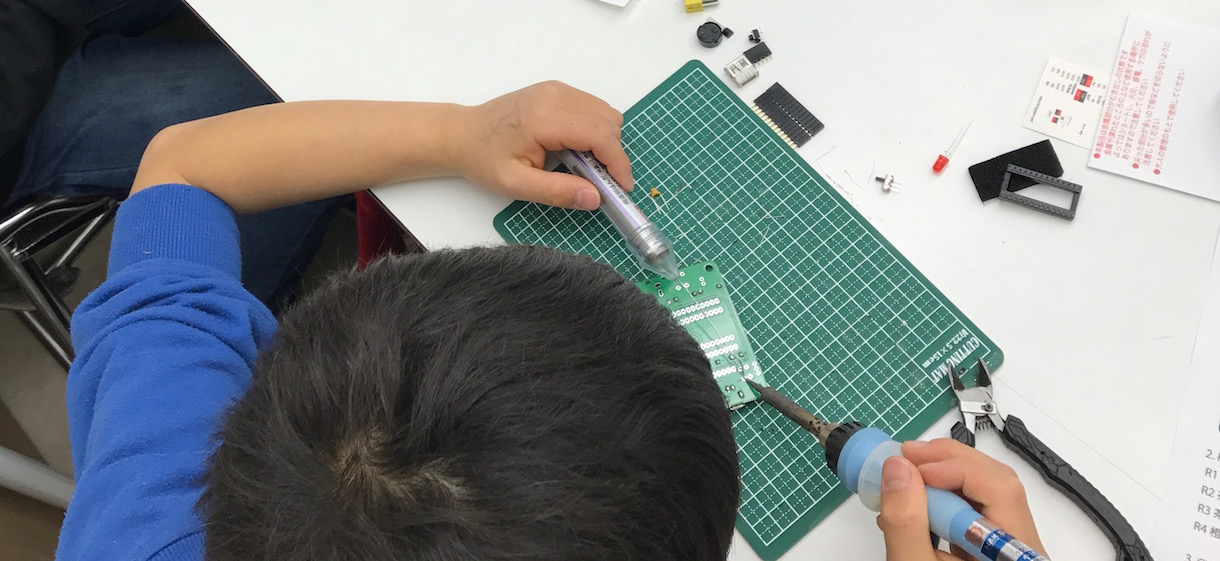8 age kid try the first soldering!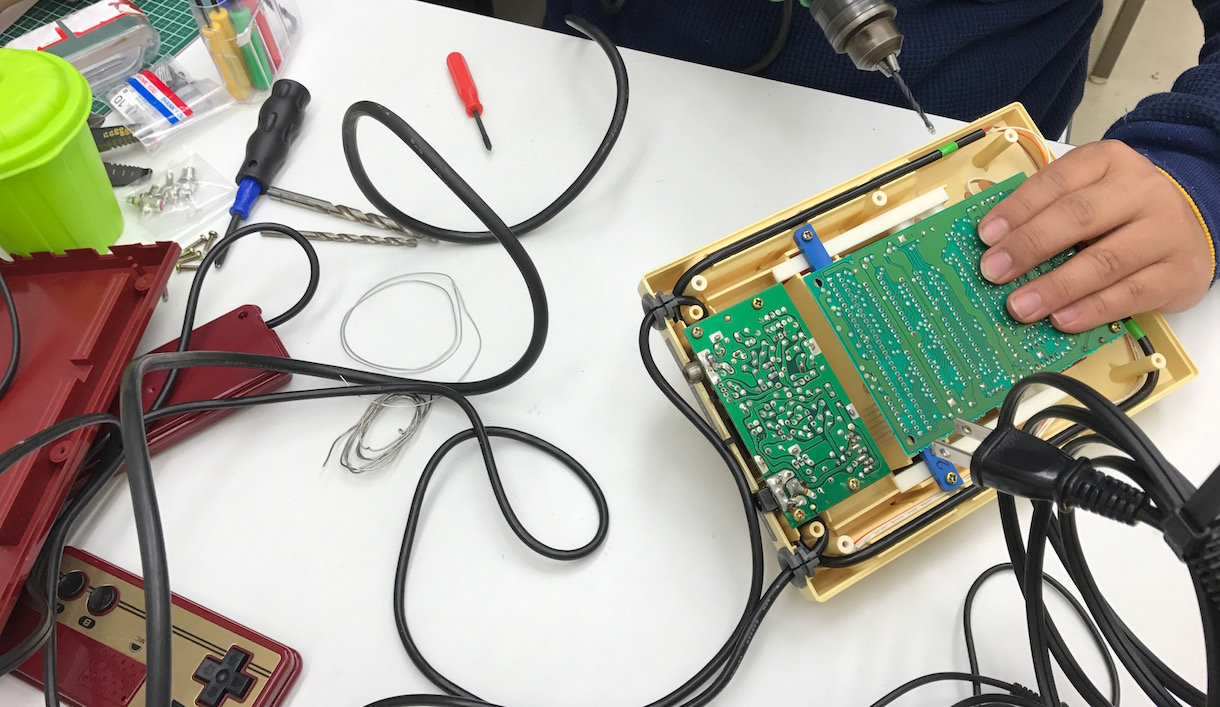Try to modify Family Computer by Nintendo!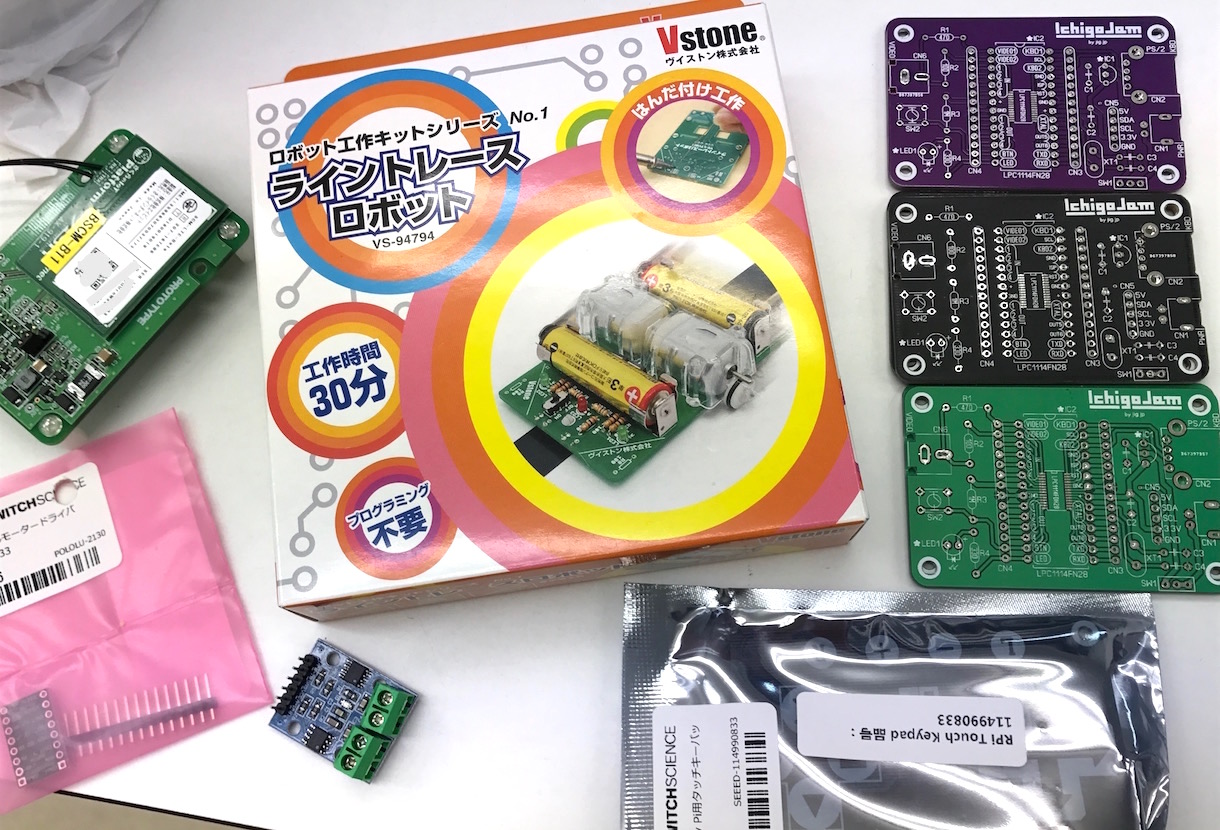Today's staffs.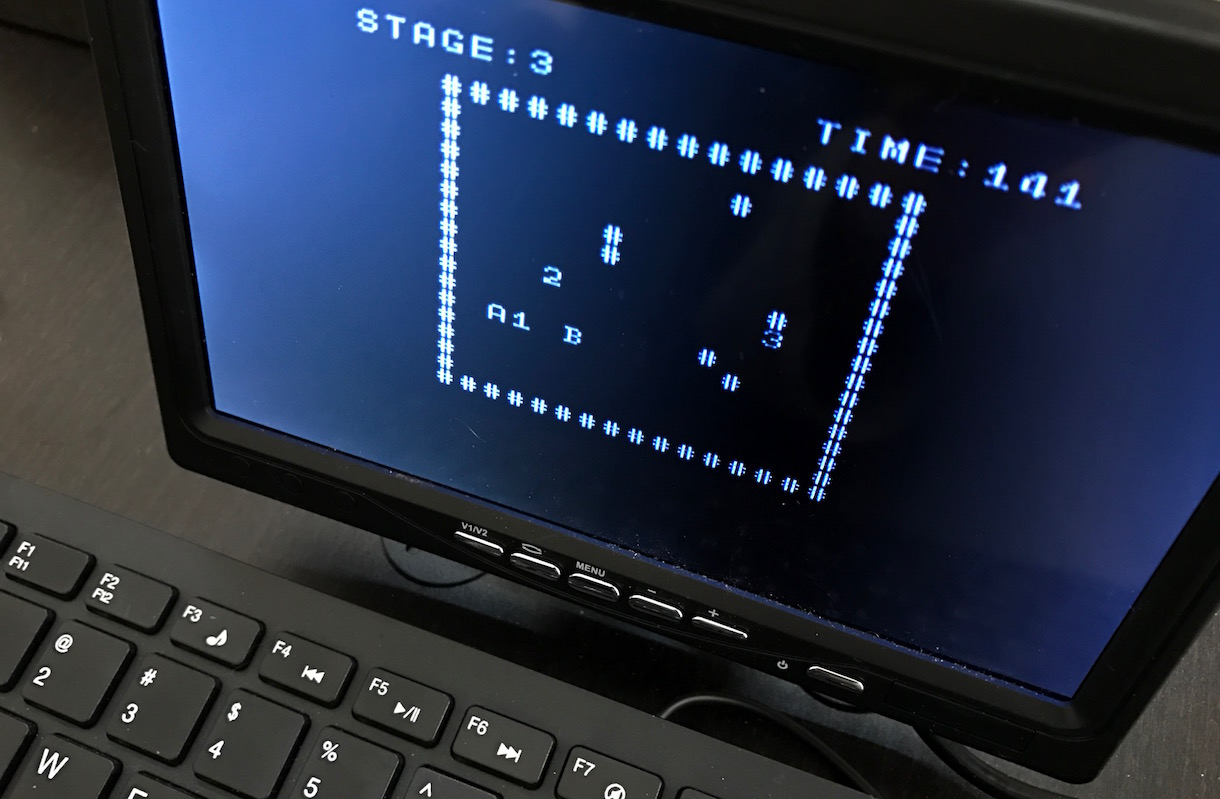This is a puzzle game "Binary Room" result of MokuMoku on my brain.
I like the game "Binary Land" puzzle game 2 game character controlled by 1 player.
Interpose by charaters A and B to get the numbers in ascending order.
Use the cusor key (A is normal movement, B is millor movement).

1 'BINARY ROOM 10 S=1 15 CLS:LC4,0:?"STAGE:";S 20 FOR I=0 TO 15 30 LC8+I,2:?"#":LC8+I,17:?"#" 50 LC8,2+I:?"#":LC23,2+I:?"#" 70 NEXT 80 SRNDS:FOR I=1 TO S*3 90 X=RND(12)+10:Y=RND(12)+4:IF SCR(X,Y) CONT 100 LCX,Y:IF I%3 ?"#" ELSE ?I/3 110 NEXT 120 X=15:Y=16:V=16:W=16:C=1:CLT 125 K=INKEY() 130 A=-(K=28)+(K=29):B=-(K=30)+(K=31) 140 IF SCR(X+A,Y+B)=0 LCX,Y:?CHR\$(0):X=X+A:Y=Y+B 150 IF SCR(V-A,W+B)=0 LCV,W:?CHR\$(0):V=V-A:W=W+B 160 LCX,Y:?"A":LCV,W:?"B" 165 LC(X+V)/2,(Y+W)/2:IF SCR()-48=C IF Y=W AND ABS(X-V)=2 OR X=V AND ABS(Y-W)=2 ?CHR\$(0);:C=C+1:IF C=S+1 LC4,20:?"CLEAR!":WAIT30:IF S=9 END ELSE S=S+1:GOTO 15 170 LC16,0:?"TIME:";TICK()/60:WAIT3:GOTO 125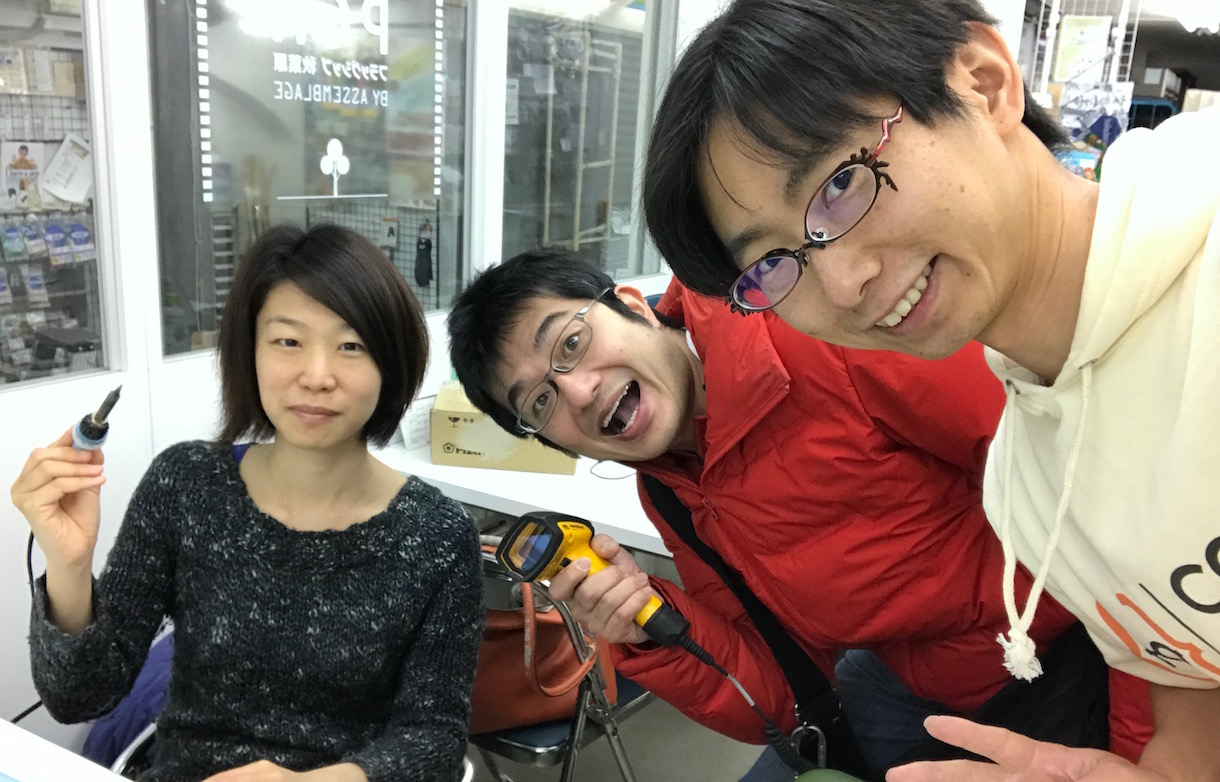See you next Moku-Moku-Meeting!CC BY / @taisukef / アイコン画像 / プロフィール画像 / 「一日一創」画像 / RSS